Next: Updating the hidden states Up: Optimising the cost function Previous: Optimising the cost function   Contents

### Updating the network weights

Assume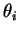is a weight in one of the MLP networks and we have evaluated the partial derivatives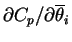and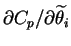. The variance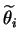is easy to update with a fixed point update rule derived by setting the derivative to zero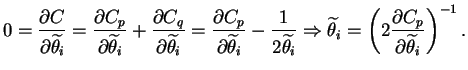(6.32)

By looking at the form of the cost function for Gaussian terms, we can find an approximation for the second derivatives with respect to the means as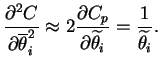(6.33)

This allows using an approximate Newton's iteration to update the mean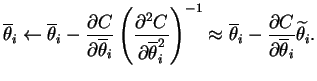(6.34)

There are some minor corrections to these update rules as explained in .

Antti Honkela 2001-05-30